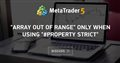# array usage , out of range error302

Hi

In line 97 and 107, out of range error is received.

This indicator drawing arrow when 2 moving averages crossed. and MAX_Profit_Up[i_Up] , MAX_Profit_Down[i_Down] should save possible profit.

For example there is 200 buy signals, I want to have each signal profit inside MAX_Profit_Up array.

I want to calculate number of profitable signals and profit average of total. first need to save profit of each signal to an array. please correct me if I'm doing wrong.

```//+------------------------------------------------------------------+
//|                                                         test.mq4 |
//+------------------------------------------------------------------+
#property strict
#property indicator_chart_window
#property indicator_buffers 2
#property indicator_color1 Blue
#property indicator_color2 Red
#property indicator_width1 3
#property indicator_width2 3
//---- input parameters

double Up[];
double Down[];

double MAX_Profit_Down[]; // Max possible profit array
double MAX_Profit_Up[]; // Max possible profit array

//+------------------------------------------------------------------+
//| Custom indicator initialization function                         |
//+------------------------------------------------------------------+

int OnInit()
{
//---- indicator buffers mapping
SetIndexBuffer(0,Up);
SetIndexBuffer(1,Down);
//---- drawing settings
SetIndexStyle(0,DRAW_ARROW);
SetIndexArrow(0,233);
SetIndexStyle(1,DRAW_ARROW);
SetIndexArrow(1,234);
//----
SetIndexEmptyValue(0,0.0);
SetIndexEmptyValue(1,0.0);
//---- name for DataWindow
SetIndexLabel(0,"Up");
SetIndexLabel(1,"Down");
//---- initialization done
return(0);
}
//+------------------------------------------------------------------+
//| Custor indicator deinitialization function                       |
//+------------------------------------------------------------------+
void OnDeinit(const int reason)
{
Comment("");
}
//+------------------------------------------------------------------+
//| Custom indicator iteration function                              |
//+------------------------------------------------------------------+

int OnCalculate(const int rates_total, // total bars
const int prev_calculated, // calculated bars by indicator
const datetime &time[],
const double &open[],
const double &high[],
const double &low[],
const double &close[],
const long &tick_volume[],
const long &volume[],
{
int i_Down=0; //signal number down
int i_Up=0; //signal number up
int n=0; // number of bars to previous signal
int status=0; // prevent repeated signals

int limit = rates_total+3;
int count=prev_calculated;
int    i;

for( i=limit-count; i>=1;i--)

{

n++;
double MA1= iMA(NULL,0,50,0,MODE_SMMA,PRICE_CLOSE,i);
double MA2= iMA(NULL,0,20,0,MODE_EMA,PRICE_CLOSE,i);

if (MA1<MA2 && (status==2||status==0))
{
status=1;
Up[i]=Low[i];
i_Up++;
MAX_Profit_Up[i_Up]= Low[iHighest(NULL,0,MODE_LOW,n,i)];
n=0;
}

if (MA1>MA2 && (status==1||status==0))
{
status=2;
Down[i]=High[i];
i_Down++;
MAX_Profit_Down[i_Down]= High[iHighest(NULL,0,MODE_HIGH,n,i)];
n=0;
}

Comment(

StringFormat(
"<<<<INFO & RATES>>>>"

"\n Number of sell signals="             "%G"
"\n =============="
"\n Number of buy signals="              "%G"
"\n Number of bars to previous Signal =" "%G"
,i_Down
,i_Up
,n

)
);

}

//----
return(0);
}
//+------------------------------------------------------------------+```Moderator
21990

KSforex:

Hi

In line 97 and 107, out of range error is received.

https://www.mql5.com/en/forum/304662#comment_10761784"array out of range" only when using "#property strict"
• 2019.02.24
• www.mql5.com
Hi This is a simple fractal indicator. MAIN QUESTION : Why getting "array out of range" error only when using "#property strict...302

Keith Watford:

https://www.mql5.com/en/forum/304662#comment_10761784

This is different problem and I can't resolve it.

I want to calculate number of profitable signals and profit average of total.

This is my code and it not work.  don't know what is the problem.

MAX_Profit_Up should return first signal max profit. But it doesn'tModerator
21990

KSforex:

This is different problem and I can't resolve it.

I want to calculate number of profitable signals and profit average of total.

This is my code and it not work.  don't know what is the problem.

MAX_Profit_Up should return first signal max profit. But it doesn't

Regarding the array out of range, it is the same problem

That is why I specifically referred to that problem

Keith Watford:

https://www.mql5.com/en/forum/304662#comment_10761784

So that people will not waste their time responding to a question that has already been answered.302

Keith Watford:

Regarding the array out of range, it is the same problem

That is why I specifically referred to that problem

So that people will not waste their time responding to a question that has already been answered.

I didn't ask what is meaning of "array out of range" !!!

People can decide themselves. I don't want you waste your time.

Thanks302

Out of range error resolved when using buffer. arrays data should be saved in memory through buffers. I'm right?!

SetIndexBuffer(2,MAX_Profit_Up);

SetIndexBuffer(3,MAX_Profit_Down);

Is it possible to do it without using SetIndexBuffer ?20823

1. ```int limit = rates_total+3;
int count=prev_calculated;
for(int i=limit-count; i>=1;i--)  ```
Invalid limits. First run count is zero so your loop starts at Bars+3 Do your lookbacks correctly.

2. ```      double MA1= iMA(NULL,0,50,0,MODE_SMMA,PRICE_CLOSE,i);
double MA2= iMA(NULL,0,20,0,MODE_EMA,PRICE_CLOSE,i);```
Your look back is max of 50 and 20.

3. ```int i_Down=0; //signal number down

if (MA1>MA2 && (status==1||status==0)){
status=2;
Down[i]=High[i];
i_Down++;
MAX_Profit_Down[i_Down]= High[iHighest(NULL,0,MODE_HIGH,n,i)]; ```
This makes no sense. If the first cross is at bar i, why would you want to mark bar one? And then the next cross at bar two?1364

This is because you declared :

```double MAX_Profit_Down[];
```

The array has no size, you cannot use it as it is, you have to resize it before using it.

ArrayResize(MAX_Profit_down, i_up +1);

i_up must be declared at a global level, ( outside oncalculate.)